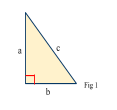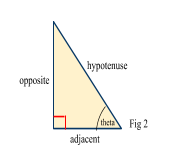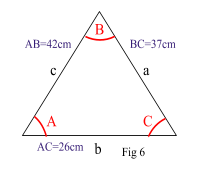# Sine and Cosine Rule

Contents

### Summary

• Pythagoras theorem: only for right angle triangles:${ a }^{ 2 }\quad +\quad { b }^{ 2 }\quad =\quad { c }^{ 2 }$
• For other triangles:
1.    Sine Rule:$\frac { a }{ sin(A) } \quad =\quad \frac { b }{ sin(B) } \quad =\quad \frac { c }{ sin(C) } \quad$

or$\frac { sin(A) }{ a } \quad =\quad \frac { sin(B) }{ b } \quad =\quad \frac { sin(C) }{ c }$

2.    Cosine Rule:${ a }^{ 2 }\quad =\quad { b }^{ 2 }\quad +\quad { c }^{ 2 }\quad -\quad 2bc\quad cos(A)$

or$cos(A)\quad =\quad \frac { { b }^{ 2 }\quad -\quad { c }^{ 2 }\quad -\quad a^{ 2 } }{ 2bc }$

• Area of a triangle :$\frac { 1 }{ 2 } absin(C)$

Let’s quickly go back and recall the pythagoras theorem. We know that for any right angle triangle, if we have got lengths of two given sides, we can find the length of the missing side using the pythagoras theorem.${ a }^{ 2 }\quad +\quad { b }^{ 2 }\quad =\quad { c }^{ 2 }$

Now, in case of a right angle triangle if we are given one length and one angle and we have to find a missing length or if we need to find a missing angle when two lengths are given we use SOH/CAH/TOA where:

SOH is$sin\theta \quad =\quad \frac { opposite }{ hypotenuse }$  shown in Fig 2CAH is$cos\theta \quad =\quad \frac { adjacent }{ hypotenuse }$  shown in Fig 3TOA is$tan\theta \quad =\quad \frac { opposite }{ adjacent }$  applies to both Fig 2 & Fig 3

However, if we want to find a missing length or an angle in a non right angle triangle we use the sine and cosine rules. Refer to Fig 4.#### Sine Rule:

We can use the sine rule to work out a missing length or an angle in a non right angle triangle, to use the sine rule we require opposites i.e one angle and its opposite length.

The sine rule is used when we are given either:

a) two angles and one side, or

b) two sides and a non-included angle.

When working out the lengths in Fig 4 :$\frac { a }{ sin(A) } \quad =\quad \frac { b }{ sin(B) } \quad =\quad \frac { c }{ sin(C) }$

When working out the angles in Fig 4:$\frac { sin(A) }{ a } \quad =\quad \frac { sin(B) }{ b } \quad =\quad \frac { sin(C) }{ c }$

Tip: Use the sine rule when a problem involves two sides and two angles

#### Cosine Rule:

The cosine rule is used when we are given either:

a) three sides and want to work out an angle or

b) two sides and the included angle.

When working out a missing side in Fig 4:${ a }^{ 2 }\quad =\quad { b }^{ 2 }\quad +\quad { c }^{ 2 }\quad -\quad 2bc\quad cos(A)$

When working out a missing angle in Fig 4:$cos(A)\quad =\quad \frac { { b }^{ 2 }\quad -\quad { c }^{ 2 }\quad -\quad a^{ 2 } }{ 2bc }$

Tip: Use the cosine rule when a problem involves three sides and one angle.

#### Area of a triangle:

We can work out the area of a triangle if its not a right angle triangle and if we don’t know the height (Fig 4), using the formula:$\frac { 1 }{ 2 } absin(C)$

#### Example #1

Q. In triangle ABC, B = 21°, C = 46°and AB = 9cm. Solve the triangle in Fig 5.Solution:

We are given two angles and one side and so the sine rule can be used.

Furthermore, since the angles in any triangle must add up to 180° then:

Angle A = 180°- (21° + 46°)
Angle A = 113°

We know that c = AB = 9cm.

Using the sine rule:$\frac { a }{ sin(113) } \quad =\quad \frac { b }{ sin(21) } \quad =\quad \frac { c }{ sin(46) }$

So:$\frac { b }{ sin(21) } \quad =\quad \frac { 9 }{ sin(46) }$$b\quad =\quad 4.484cm$

Similarly:$\frac { a }{ sin(113) } \quad =\quad \frac { 9 }{ sin(46) }$$a\quad =\quad 11.517cm$

We have now worked out all the missing angle and the missing lengths of the triangle in Fig 5.

#### Example #2

Q. In triangle ABC, AB = 42cm, BC = 37cm and AC =26cm. Solve the triangle in Fig 6.Solution:

We are given three sides of the triangle and so the cosine rule can be used.

Writing a = 37, b = 26 and c = 42 we have:${ a }^{ 2 }\quad =\quad { b }^{ 2 }\quad +\quad { c }^{ 2 }\quad -\quad 2bc\quad cos(A)$

Substituting values in the above equation:$37^{ 2 }\quad =\quad { 26 }^{ 2 }\quad +\quad { 42 }^{ 2 }\quad -\quad 2(26)(42)cos(A)$$cos(A)\quad =\quad \frac { 1071 }{ 2184 } \quad =\quad 0.4904$$A\quad =\quad { cos }^{ -1 }(0.4904)\quad =\quad 60.63$°

You should apply the same technique to verify that B = 37.76° and C = 81.61°. You should also check that the angles you obtain add up to 180◦.